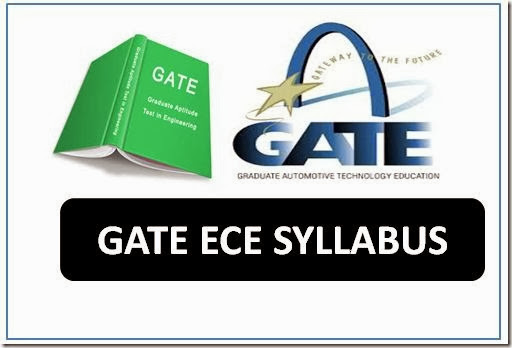# GATE syllabus for ECE 2021 download in pdf formatGate is the one of the most competitive exam in India to took PG-M.tech or M.S or Phd admission in top most colleges like IIT,NIT,Top universities,Bits pilani and it also useful for PSU’S jobs… here I am giving latest GATE syllabus for ece 2021 in pdf format which we are expecting similar to gate 2020 syllabus.

The Gate exam is also used to get a job in PSU's, the gate score is very useful for your career. In addition to the below syllabus there are two more sections 1: general English  and 2. aptitude

ENGINEERING MATHEMATICS:
Linear Algebra: Vector space, basis, linear dependence and independence, matrix algebra, eigen values and eigen vectors, rank, solution of linear equations – existence and uniqueness.

Calculus: Mean value theorems, theorems of integral calculus, evaluation of definite and improper integrals, partial derivatives, maxima and minima, multiple integrals, line, surface and volume integrals, Taylor series.

Differential Equations: First order equations (linear and nonlinear), higher order linear differential equations, Cauchy's and Euler's equations, methods of solution using variation of parameters, complementary function and particular integral, partial differential equations, variable separable method, initial and boundary value problems.

Vector Analysis: Vectors in plane and space, vector operations, gradient, divergence and curl, Gauss's, Green's and Stoke's theorems.

Complex Analysis: Analytic functions, Cauchy's integral theorem, Cauchy's integral formula; Taylor's and Laurent's series, residue theorem.

Numerical Methods: Solution of nonlinear equations, single and multi-step methods for differential equations, convergence criteria.

Probability and Statistics: Mean, median, mode and standard deviation; combinatorial probability, probability distribution functions - binomial, Poisson, exponential and normal; Joint and conditional probability; Correlation and regression analysis.
##### ELECTRONICS AND COMMUNICATION ENGINEERING
Networks, Signals and Systems :Network solution methods: nodal and mesh analysis; Network theorems: superposition, Thevenin and Norton’s, maximum power transfer; Wye‐Delta transformation; Steady state sinusoidal analysis using phasors; Time domain analysis of simple linear circuits; Solution of network equations using Laplace transform; Frequency domain analysis of RLC circuits; Linear 2‐port network parameters: driving point and transfer functions; State equations for networks. Continuous-time signals: Fourier series and Fourier transform representations, sampling theorem and applications;
Discrete-time signals: discrete-time Fourier transform (DTFT), DFT, FFT, Z-transform, interpolation of discrete-time signals; LTI systems: definition and properties, causality, stability, impulse response, convolution, poles and zeros, parallel and cascade structure, frequency response, group delay, phase delay, digital filter design techniques.

Electronic Devices : Energy bands in intrinsic and extrinsic silicon; Carrier transport: diffusion current, drift current, mobility and resistivity; Generation and recombination of carriers; Poisson and continuity equations; P-N junction, Zener diode, BJT, MOS capacitor, MOSFET, LED, photo diode and solar cell; Integrated circuit fabrication process: oxidation, diffusion, ion implantation, photolithography and twin-tub CMOS process.

Analog Circuits: Small signal equivalent circuits of diodes, BJTs and MOSFETs; Simple diode circuits: clipping, clamping and rectifiers; Single-stage BJT and MOSFET amplifiers: biasing, bias stability, mid-frequency small signal analysis and frequency response; BJT and MOSFET amplifiers: multi-stage, differential, feedback, power and operational; Simple op-amp circuits; Active filters; Sinusoidal oscillators: criterion for oscillation, single-transistor and opamp configurations; Function generators, wave-shaping circuits and 555 timers; Voltage reference circuits; Power supplies: ripple removal and regulation.

Digital circuits: Number systems; Combinatorial circuits: Boolean algebra, minimization of functions using Boolean identities and Karnaugh map, logic gates and their static CMOS implementations, arithmetic circuits, code converters, multiplexers, decoders and PLAs; Sequential circuits: latches and flip‐flops, counters, shift‐registers and finite state machines; Data converters: sample and hold circuits, ADCs and DACs; Semiconductor memories: ROM, SRAM, DRAM; 8-bit microprocessor (8085): architecture, programming, memory and I/O interfacing.

Control Systems: Basic control system components; Feedback principle; Transfer function; Block diagram representation; Signal flow graph; Transient and steady-state analysis of LTI systems; Frequency response; Routh-Hurwitz and Nyquist stability criteria; Bode and root-locus plots; Lag, lead and lag-lead compensation; State variable model and solution of state equation of LTI systems.

Communications: Random processes: autocorrelation and power spectral density, properties of white noise, filtering of random signals through LTI systems; Analog communications: amplitude modulation and demodulation, angle modulation and demodulation, spectra of AM and FM, superheterodyne receivers, circuits for analog communications; Information theory: entropy, mutual information and channel capacity theorem; Digital communications: PCM, DPCM, digital modulation schemes, amplitude, phase and frequency shift keying (ASK, PSK, FSK), QAM, MAP and ML decoding, matched filter receiver, calculation of bandwidth, SNR and BER for digital modulation; Fundamentals of error correction, Hamming codes; Timing and frequency synchronization, inter-symbol interference and its mitigation; Basics of TDMA, FDMA and CDMA.

Electromagnetics: Electrostatics; Maxwell’s equations: differential and integral forms and their interpretation, boundary conditions, wave equation, Poynting vector; Plane waves and properties: reflection and refraction, polarization, phase and group velocity, propagation through various media, skin depth; Transmission lines: equations, characteristic impedance, impedance matching, impedance transformation, S-parameters, Smith chart; Waveguides: modes, boundary conditions, cut-off frequencies, dispersion relations; Antennas: antenna types, radiation pattern, gain and directivity, return loss, antenna arrays; Basics of radar; Light propagation in optical fibers.

I hope this ece syllabus will help you in the preparation of GATE 2021 exam. For more updates please subscribe our blog.

#### 1 comment :

1.Hello Friends,Is it necessary to join gate online tests series?